# Texas Go Math Grade 3 Lesson 3.4 Answer Key Model Equivalent Fractions

Refer to our Texas Go Math Grade 3 Answer Key Pdf to score good marks in the exams. Test yourself by practicing the problems from Texas Go Math Grade 3 Lesson 3.4 Answer Key Model Equivalent Fractions.

## Texas Go Math Grade 3 Lesson 3.4 Answer Key Model Equivalent Fractions

Essential Question
How can you use models to find equivalent fractions?
Two fractions are equivalent (equal) if they are of the same size or are at the same point on a number line.

Investigate
Materials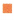sheet of papercrayon or color pencil

Two or more fractions that name the same amount are called equivalent fractions. You can use a sheet of paper
to model fractions equivalent to $$\frac{1}{2} \text {. }$$

A. First, fold a sheet of paper into two equal parts. Open the paper and count the parts.
There are 2 equal parts. Each part is $$\frac{1}{2}$$ of the paper.
Shade one of the halves. Write $$\frac{1}{2}$$ on each of the halves.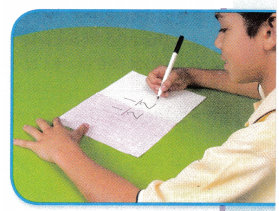B. Next, fold the paper in half two times. Open the paper.
Now there are 4 equal parts. Each part is $$\frac{1}{4}$$ of the paper.
Write $$\frac{1}{4}$$ on each of the fourths.
Look at the shaded parts. $$\frac{1}{2}$$ = $$\frac{2}{4}$$C. Last, fold the paper in half three times.
Now there are 8 equal parts. Each part is $$\frac{1}{8}$$ of the paper.
Write $$\frac{1}{8}$$ on each of the eighths.
So,
$$\frac{1}{2}$$ = $$\frac{4}{8}$$
Find the fractions equivalent to $$\frac{1}{2}$$ on your paper.
So,
$$\frac{1}{2}$$, $$\frac{2}{4}$$, and $$\frac{4}{8}$$ are equivalent.

Make Connections

You can use a number line to find equivalent fractions
Find a fraction equivalent to $$\frac{2}{3}$$.

Materials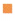fraction strips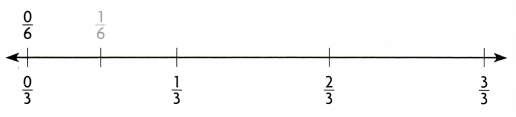Math Talk
Mathematical Processes
Explain how the number of sixths in a distance on the number line is related to the number of thirds in the same distance.
The given number line is:Now,
We know that,
$$\frac{1}{6}$$ = $$\frac{1}{2}$$ × $$\frac{1}{3}$$
So,
We will obtain the sixths by dividing $$\frac{1}{3}$$ into 2 parts
Hence, from the above,
We can conclude that
The number of sixths in a distance on the number line is $$\frac{1}{2}$$ times the number of thirds in the same distance

Step 1
Draw a point on the number line to represent the distance $$\frac{2}{3}$$.
The given number line is:Hence,
The representation of $$\frac{2}{3}$$ on the given number line is:Step 2
Use fraction strips to divide the number line into sixths. At the end of each strip, draw a mark on the number line and label the marks to show sixths.
The given number line is:Hence,
The representation of the sixths on the given number line is:Step 3
Identify the fraction that names the same point as $$\frac{2}{3}$$.
So, $$\frac{2}{3}$$ = $$\frac{4}{6}$$

Share and Show

Shade the model. Then divide the pieces to find the equivalent fraction.

Question 1.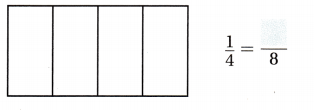The representation of the model with the original and equivalent fractions is: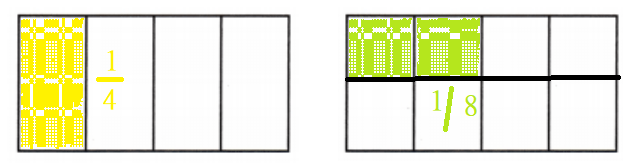Hence, from the above,
We can conclude that
$$\frac{1}{4}$$ = $$\frac{2}{8}$$

Question 2.The representation of the model with the original and equivalent fractions is:Hence, from the above,
We can conclude that
$$\frac{2}{3}$$ = $$\frac{4}{6}$$

Use fraction strips or the number line to find the equivalent fraction.

Question 3.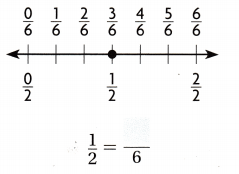The given number line is:Now,
From the given number line,
We can observe that
$$\frac{1}{2}$$ = $$\frac{3}{6}$$
Hence, from the above,
We can conclude thatQuestion 4.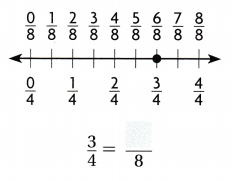The given number line is: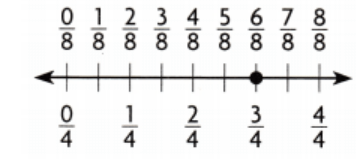Now,
From the given number line,
We can observe that
$$\frac{3}{4}$$ = $$\frac{6}{8}$$
Hence, from the above,
We can conclude that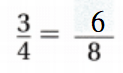Problem Solving

Use the pictures to solve 5-9.

Question 5.
H.O.T. Multi-Step Mrs. Akers bought three sandwiches that were the same size. She cut the first one into thirds. She cut the second one into fourths and the third one into sixths. Marian ate 2 pieces of the first sandwich. Jason ate 2 pieces of the second sandwich. Marcos ate 3 pieces of the third sandwich. Which children ate the same amount of a sandwich?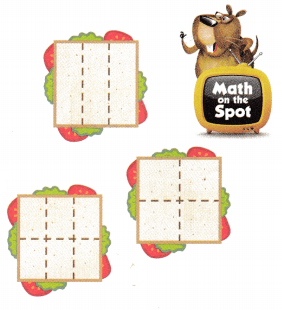The first sandwich was cut into 3 parts.
Manan ate 2 pieces of the sandwich. Shade the part Manan ate.Manan ate $$\frac{2}{3}$$ of the first sandwich.

The second sandwich was cut into 4 pieces
Jason ate 2 pieces of the sandwich. Shade the part Jason ate.Jason ate$$\frac{2}{4}$$ of the second sandwich.

The third sandwich was cut into 6 pieces.
Marcos ate 3 pieces of the sandwich. Shade the part Marcos ate.Marcos ate $$\frac{3}{6}$$ of the third sandwich.

Question 6.
Are all the fractions equivalent?
From the above problem,
The fractions we obtained are:
$$\frac{2}{3}$$, $$\frac{2}{4}$$, and $$\frac{3}{6}$$
So,
From the above fractions,
We can observe that
$$\frac{2}{4}$$ = $$\frac{3}{6}$$ = $$\frac{1}{2}$$
Hence, from the above,
We can conclude that
All the fractions are not equivalent

Question 7.
Which fractions are equivalent?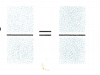From the above problem,
The fractions we obtained are:
$$\frac{2}{3}$$, $$\frac{2}{4}$$, and $$\frac{3}{6}$$
So,
From the above fractions,
We can observe that
$$\frac{2}{4}$$ = $$\frac{3}{6}$$ = $$\frac{1}{2}$$
Hence, from the above,
We can conclude that
The fractions that are equivalent is:
$$\frac{2}{4}$$ = $$\frac{3}{6}$$

Question 8.
So, __ and ___ ate the same amount of a sandwich.
So,
Jason and Marcos ate the same amount of a sandwich

Question 9.
Analyze What if Mrs. Akers cut a fourth sandwich into sixths? What fraction is equivalent to the amount Manan ate?
It is given that
Mrs. Akers cut the fourth sandwich into sixths
So,
The amount of each sandwich Mrs. Akers will cut from the fourth sandwich = $$\frac{1}{6}$$
Now,
From the above problem,
We can observe that
The fraction of the sandwich that Marian ate is: $$\frac{2}{3}$$
Hence, from the above,
We can conclude that
The fraction of the sandwich that is equivalent to Marian ate is: $$\frac{4}{6}$$ (or) $$\frac{6}{9}$$

Fill in the bubble for the correct answer choice.

Question 10.
Three brothers want an equal share of an apple. Each will get $$\frac{1}{3}$$ of the apple. They cut the apple into thirds and then into sixths. How many $$\frac{1}{6}$$ parts are equal to $$\frac{1}{3}$$ ?
(A) 1
(B) 3
(C) 2
(D) 6
It is given that
Three brothers want an equal share of an apple. Each will get $$\frac{1}{3}$$ of the apple. They cut the apple into thirds and then into sixths
Now,
We know that,
$$\frac{1}{6}$$ = $$\frac{1}{2}$$ × $$\frac{1}{3}$$
So,
$$\frac{1}{3}$$ = 2 × $$\frac{1}{6}$$
Hence, from the above,
We can conclude that
The number of $$\frac{1}{6}$$ parts that are equal to $$\frac{1}{3}$$ is: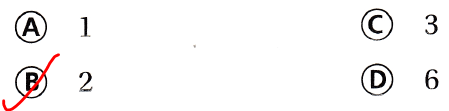Question 11.
Use Tools Kenny ate $$\frac{3}{4}$$ of an orange. Which fraction is equivalent to $$\frac{3}{4}$$?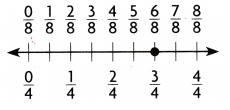(A) $$\frac{1}{8}$$
(B) $$\frac{2}{8}$$
(C) $$\frac{3}{8}$$
(D) $$\frac{6}{8}$$
It is given that
Kenny ate $$\frac{3}{4}$$ of an orange
Now,
The given number line is:Now,
From the above number line,
We can observe that
$$\frac{3}{4}$$ = $$\frac{6}{8}$$
Hence, from the above,
We can conclude that
The fraction that is equivalent to $$\frac{3}{4}$$ is:Question 12.
Use Diagrams Multi-Step Look at the pizza at the right Which shows an equivalent fraction of eaten pizza?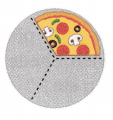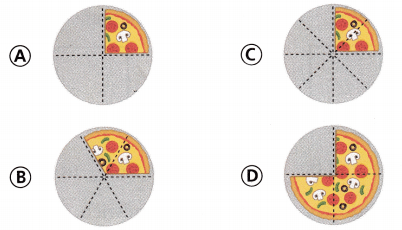The given figure that represents thee aten pizza is:Now,
From the above figure,
We can observe that
The fraction of the eaten pizza = $$\frac{1}{3}$$
So,
The fractions that are equivalent to $$\frac{1}{3}$$ are: latex]\frac{2}{6}[/latex] (or) $$\frac{3}{9}$$
Hence, from the above,
We can conclude that
The figure that shows an equivalent fraction of eaten pizza is:Texas Test Prep

Question 13.
Maria has $$\frac{1}{2}$$ of an obstacle course left to finish. What fraction is equivalent to $$\frac{1}{2}$$?(A) $$\frac{2}{1}$$
(B) $$\frac{5}{6}$$
(C) $$\frac{3}{6}$$
(D) $$\frac{2}{6}$$
It is given that
Maria has $$\frac{1}{2}$$ of an obstacle course left to finish
Now,
The given number line is:Now,
From the above number line,
We can observe that
$$\frac{1}{2}$$ = $$\frac{3}{6}$$
Hence, from the above,
We can conclude that
The fraction that is equivalent to $$\frac{1}{2}$$ is: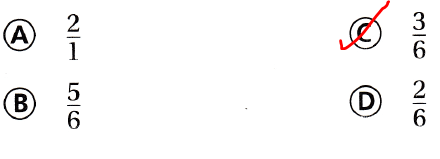### Texas Go Math Grade 3 Lesson 3.4 Homework and Practice Answer Key

Shade the model. Then divide the pieces to find the equivalent fraction.

Question 1.The representation of the model with the original and equivalent fractions is:Hence, from the above,
We can conclude that
$$\frac{3}{4}$$ = $$\frac{6}{8}$$

Question 2.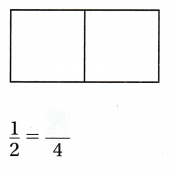The representation of the model with the original and equivalent fractions is:Hence, from the above,
We can conclude that
$$\frac{1}{2}$$ = $$\frac{2}{4}$$

Question 3.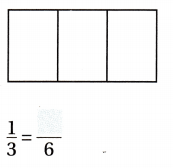The representation of the model with the original and equivalent fractions is:Hence, from the above,
We can conclude that
$$\frac{1}{3}$$ = $$\frac{2}{6}$$

Question 4.The representation of the model with the original and equivalent fractions is: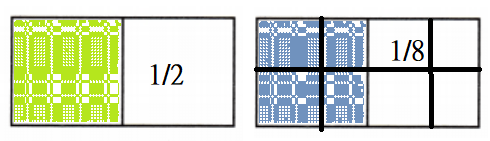Hence, from the above,
We can conclude that
$$\frac{1}{2}$$ = $$\frac{4}{8}$$

Problem Solving

Question 5.
Jason cut a sandwich into three equal pieces and ate two of the pieces. Carl cut a same-size sandwich into six pieces. Carl ate the same fraction of his sandwich as Jason did. What fraction of the sandwich did Carl eat?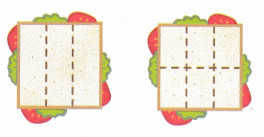It is given that
Jason cut a sandwich into three equal pieces and ate two of the pieces. Carl cut a same-size sandwich into six pieces. Carl ate the same fraction of his sandwich as Jason did
Now,
According to the given information,
The number of pieces Jason ate the sandwich = $$\frac{2}{3}$$
Now,
Let the number of pieces Carl ate be: x pieces
So,
The number of pieces Carl ate the sandwich = $$\frac{x}{6}$$
So,
$$\frac{2}{3}$$ = $$\frac{x}{6}$$
x = 4 pieces
Hence, from the above,
We can conclude that
The fraction of the sandwich did Carl eat is: $$\frac{4}{6}$$

Lesson Check

Fill in the bubble completely to show your answer. Use the number lines for 6-7.

Question 6.
After finishing $$\frac{1}{4}$$ of the soccer practice, players take a water break. Which fraction is equivalent to $$\frac{1}{4}$$?(A) $$\frac{0}{8}$$
(B) $$\frac{2}{8}$$
(C) $$\frac{6}{8}$$
(D) $$\frac{3}{8}$$
It is given that
After finishing $$\frac{1}{4}$$ of the soccer practice, players take a water break
Now,
The given number line is:Now,
From the above number line,
We can observe that
$$\frac{1}{4}$$ = $$\frac{2}{8}$$
Hence, from the above,
We can conclude that
The fraction that is equivalent to $$\frac{1}{4}$$ is:Question 7.
Carlos practiced the piano for $$\frac{2}{3}$$ hour. Which fraction is equivalent to $$\frac{2}{3}$$?(A) $$\frac{1}{6}$$
(B) $$\frac{3}{6}$$
(C) $$\frac{1}{6}$$
(D) $$\frac{4}{6}$$
It is given that
Carlos practiced the piano for $$\frac{2}{3}$$ hour
Now,
The given number line is:Now,
From the above number line,
We can observe that
$$\frac{2}{3}$$ = $$\frac{4}{6}$$
Hence, from the above,
We can conclude that
The fraction that is equivalent to $$\frac{2}{3}$$ is: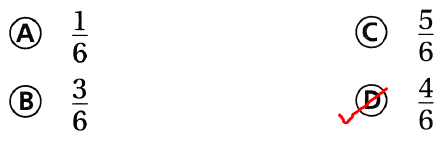Question 8.
Multi-Step Look at the circle below. Which model shows an equivalent fraction?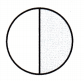The given figure is:Now,
From the above model,
We can observe that
The fraction of the shaded part in the given figure = $$\frac{1}{2}$$
So,
The fraction that is equivalent to $$\frac{1}{2}$$ is: $$\frac{2}{4}$$ (or) $$\frac{3}{6}$$
Hence, from the above,
We can conclude that
The model that shows the equivalent fraction for the given model is: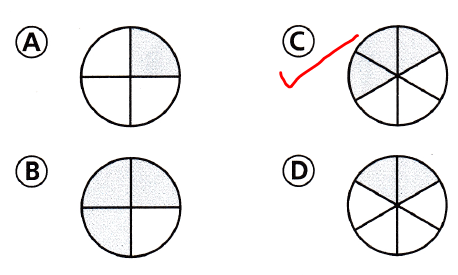Question 9.
Multi-Step A banner is divided into six equal parts and four parts are blue. Which fraction is equivalent to the blue parts of the banner?(A) $$\frac{1}{3}$$
(B) $$\frac{2}{4}$$
(C) $$\frac{3}{4}$$
(D) $$\frac{2}{3}$$
It is given that
A banner is divided into six equal parts and four parts are blue
Now,
The given model is:Now,
According to the given information,
The fraction that is equivalent to the blue parts of the banner = (The number of parts that are blue) ÷ (The total number of parts)
= $$\frac{4}{6}$$
= $$\frac{2}{3}$$
Hence, from the above,
We can conclude that
The fraction that is equivalent to the blue parts of the banner is: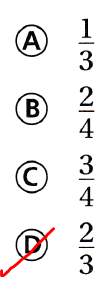Scroll to Top